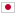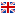Contest Duration: - (local time) (300 minutes)
B - Tournament Chart/Time Limit: 2 sec / Memory Limit: 512 MB

### Problem Statement

In 21XX, an annual programming contest, Japan Algorithmist GrandPrix (JAG) has become one of the most popular mind sports events.

JAG is conducted as a knockout tournament. This year, $N$ contestants will compete in JAG. A tournament chart is represented as a string. [[a-b]-[c-d]] is an easy example. In this case, there are 4 contestants named a, b, c, and d, and all matches are described as follows:

• Match 1 is the match between a and b.
• Match 2 is the match between c and d.
• Match 3 is the match between [the winner of match 1] and [the winner of match 2].

More precisely, the tournament chart satisfies the following BNF:

• <winner> ::= <person> | "[" <winner> "-" <winner> "]"
• <person> ::= "a" | "b" | "c" | ... | "z"

You, the chairperson of JAG, are planning to announce the results of this year's JAG competition. However, you made a mistake and lost the results of all the matches. Fortunately, you found the tournament chart that was printed before all of the matches of the tournament. Of course, it does not contains results at all. Therefore, you asked every contestant for the number of wins in the tournament, and got $N$ pieces of information in the form of "The contestant $a_i$ won $v_i$ times".

Now, your job is to determine whether all of these replies can be true.

### Input

The input consists of a single test case in the format below.

$S$ $a_1$ $v_1$ $\vdots$ $a_N$ $v_N$

$S$ represents the tournament chart. $S$ satisfies the above BNF. The following $N$ lines represent the information of the number of wins. The $(i+1)$-th line consists of a lowercase letter $a_i$ and a non-negative integer $v_i$ ($v_i \le 26)$ separated by a space, and this means that the contestant $a_i$ won $v_i$ times. Note that $N$ ($2 \le N \le 26$) means that the number of contestants and it can be identified by string $S$. You can assume that each letter $a_i$ is distinct. It is guaranteed that $S$ contains each $a_i$ exactly once and doesn't contain any other lowercase letters.

### Output

Print Yes in one line if replies are all valid for the tournament chart. Otherwise, print No in one line.

### Sample Input 1

[[m-y]-[a-o]]
o 0
a 1
y 2
m 0


### Output for Sample Input 1

Yes


### Sample Input 2

[[r-i]-[m-e]]
e 0
r 1
i 1
m 2


### Output for Sample Input 2

No